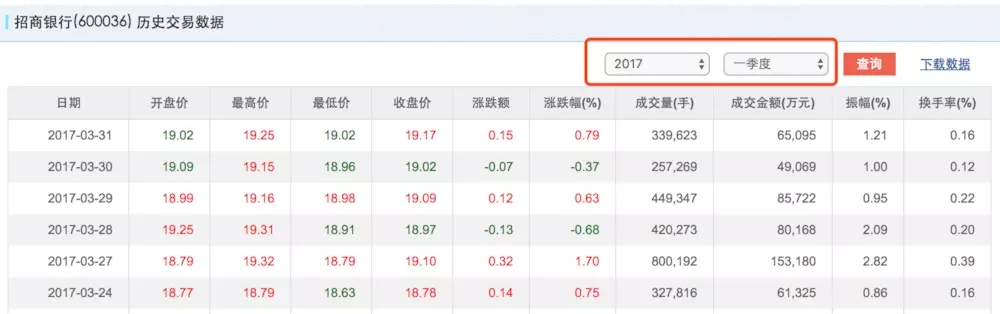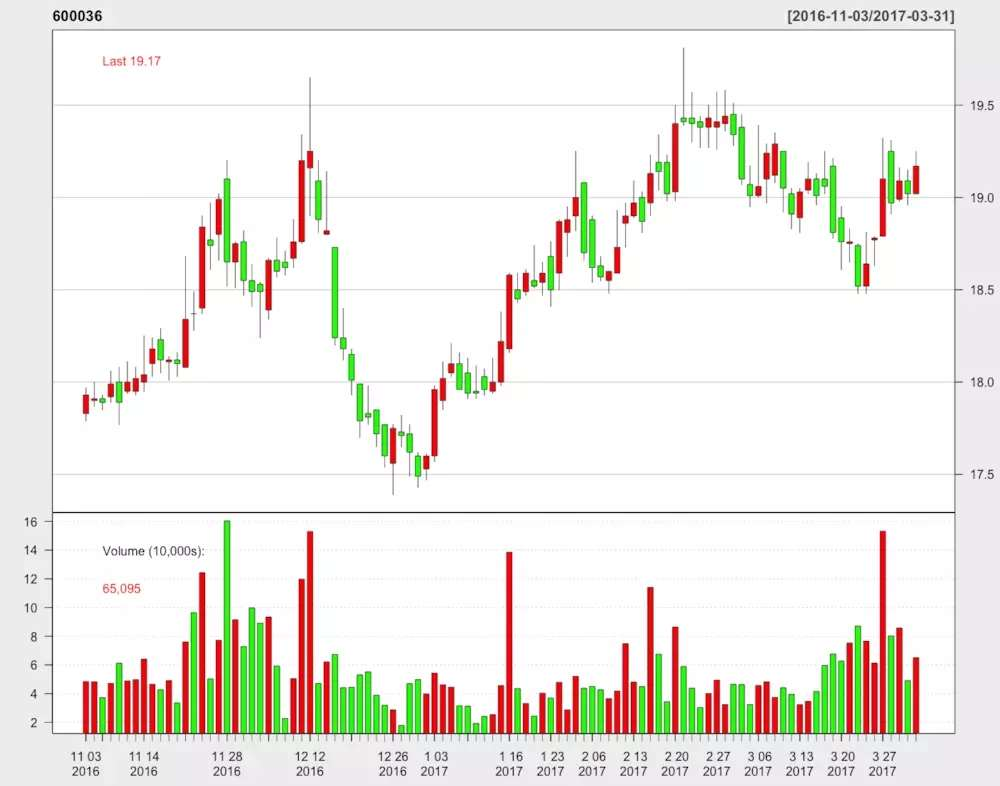# 使用Python画股票的K线图的方法步骤

更新时间：2019年06月28日 10:01:24   作者：allenmagic我要评论

1、构建爬虫获取股票价格数据

```import re,urllib2,time,csv,datetime
import matplotlib as mpl
import matplotlib.pyplot as plt
import matplotlib.finance as mpf
import matplotlib.dates as mpd```

```t = time.localtime() # 获取当前的本地时间
year = range(t,1989,-1) # 设定年度范围，从当前年度至沪市开市的年份倒序生成
season = range(4,0,-1)  # 生成季度的数据列表，从4季度到1季度倒序生成``````def getData(url):
request = urllib2.Request(url)
response = urllib2.urlopen(request)

ta = re.findall(pattern, str(content))
pattern1 = re.compile("<td class='cGreen'>")
pattern2 = re.compile("<td class='cRed'>")
pattern3 = re.compile(",")
tab1 = re.sub(pattern1,"<td>",str(ta))
tab2 = re.sub(pattern2,"<td>",str(tab1))
tab = re.sub(pattern3, "", str(tab2))

if len(tab) == 0:
data = []
else:
pattern3 = re.compile('<td>(.*?)</td>')
data = re.findall(pattern3, str(tab))

for d in data:
if d == '':
data.remove('')

return data

```

2. 匹配<td class='cGreen'>、<td class='cRed'>并使用<td>替换，因为这两个字符串会影响后续的匹配数据，现行替换掉可以更方便的匹配到需要的数据
3. 替换到千分位","号，因为Python和R并不会识别有千分位号的数据，所以我们要将数据转换为非千分位的数据。
4. tab是按照要求最后获取的包含数据和文本的原始内容
5. 用if函数来获取除文本的数据，因为如果year和season超过了当前的界限，会返回空的tab，所以我们在这里进行判断，如果少了这个判断，会报出index error。这个if函数表示了如果tab为空，data也是个空的列表，如果tab不为空，那么根据pattern3返回需要的数据至data列表
6. 用一个for循环来遍历data列表，删除空白的内容（其实这一步不需要，因为在if中已经剔除了空的内容。

```def get_stock_price(code):
url2 = ".html?year="
url3 = "&season="
urllist = []
for k in year:
for v in season:
urllist.append(url1+str(code)+url2+str(k)+url3+str(v))

price = []
for url in urllist:
price.extend(getData(url))
return price
```

1. 根据code构建其股票数据的页面的url列表
2. 使用getData(url）函数和for循环，返回所有的历史数据

```# get all histrocial data include all price and others
price = get_stock_price(600036)```

2、保存数据

```writer = csv.writer(file("stock.csv",'wb'))
writer.writerow(['Date','Open','High','Low','Close','Volume'])
pr = []
for i in range(0,len(price),11):
pr.extend([[price[i],price[i+1],price[i+2],price[i+3],price[i+4],price[i+8]]])

for prl in pr:
writer.writerow(prl)

```

```# get the number for date by date2num
def Date_no(strdate):
t = time.strptime(strdate, "%Y-%m-%d")
y,m,d = t[0:3]
d = datetime.date(y, m, d)
n = mpd.date2num(d)

return n

# get the price data
pr = []
for i in range(0,len(price),11):
pr.extend([[
Date_no(price[i])
,float(price[i+1])
,float(price[i+2])
,float(price[i+3])
,float(price[i+4])
,float(price[i+8])]]
)

```

```library(quantmod)

rm(list = ls())
setwd("~/GitHub/index/")

n <- nrow(price)
m <- nrow(price)-100

#pdf(file = "k.pdf")
chartSeries(price[c(m:n)],theme = chartTheme("white"),up.col = "red",dn.col = "green",name = "600036",time.scale = 0.5,line.type = "l",bar.type = "ohlc",major.ticks='auto', minor.ticks=TRUE)
#dev.off()

```R中可以使用quantmod包中的chartSeries函数画出K线图，具体的使用方法可以参考chartSeries参考文档

```quotes = pr[0:80]

print(quotes)

fig,ax = plt.subplots(figsize=(30,6))
mpf.candlestick_ohlc(ax,quotes,width=0.4,colorup='r',colordown='g')
plt.grid(False)
ax.xaxis_date()
ax.autoscale_view()
plt.setp(plt.gca().get_xticklabels(), rotation=30)
plt.show()

```

K线效果图如下：